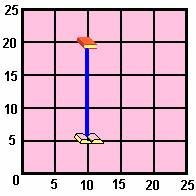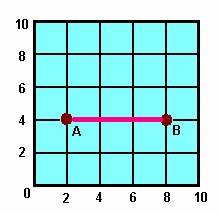Definition Of Distance

he Distance between two points is defined as the shortest length between them.

Distance is a scalar quantity.

Example Of DistanceThe line joining the open and closed books is a vertical line.
The length of a vertical line-segment on a coordinate plane equals the difference between the y-coordinates.
So, the distance between the two books = 20 - 5 = 15 units.

Solved Example on Distance

Ques: Find the distance between the two points A and B.A. 6 units
B. 8 units
C. 4 units
D. 2 units